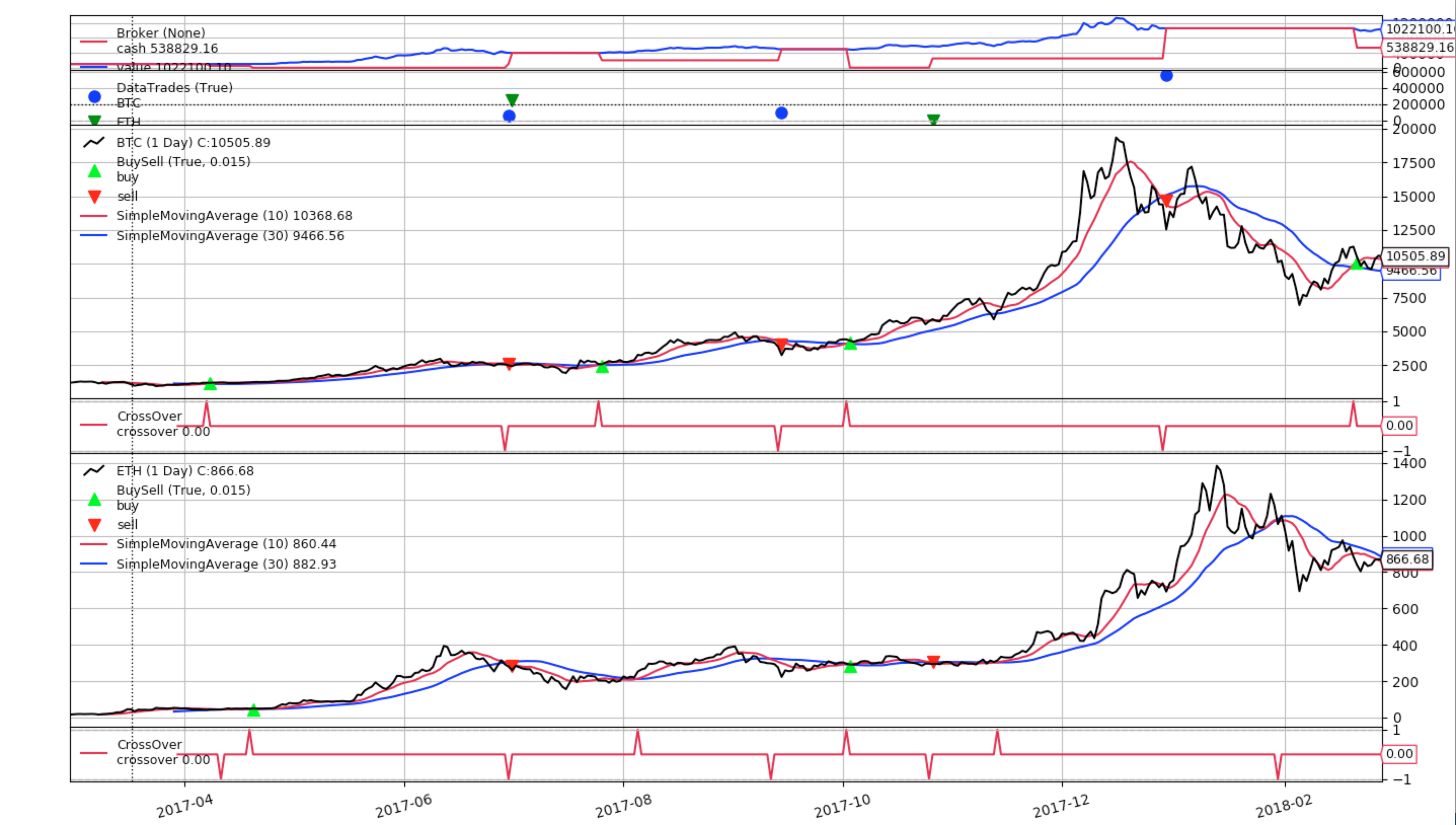For code/output blocks: Use ``` (aka backtick or grave accent) in a single line before and after the block. See: http://commonmark.org/help/

# Why don't the orders happen for every signal?

• I'm using the SMA Crossover strategy with bitcoin and ether prices. As you can see below, there are two graphs, one for each currency. The problem is in the lower graph (ether): not every signal results in a buy / sell. This is my `next` method of the strategy:

``````    def next(self):
stake = 1 / len(self.datas)
for i, data in enumerate(self.datas):
signal = self.signals[i]
if signal > 0:
self.order_target_percent(target=stake, data=data)
elif signal < 0:
self.order_target_percent(target=0.0, data=data)
``````

How come this strategy is not creating an order for every signal?• Your strategy may not have enough money to buy. When you issue the order, the known price is the `close` price. If the `open` price gaps up/down, you won't get in the market.

(In any case: we see no graphs)

• Modified the original post to include the graph.

Looks like you're right. My previous code tried to order target percentage of 50% for both ether and bitcoin. After I reduced the percentage to 25% I was able to fill in every order.

Why exactly does this happen? Is it because the following happens (giving a made-up example)?

2. Order 50% of bitcoin, means I trade 50k USD for bitcoin.
3. Bitcoin price rises after some time, my portfolio is worth 110k (50k left in USD + 60k worth of bitcoin).
4. Want to buy 50% ether, that would be 55k, but we only have 50k USD.

But if that was the case, then how would it be possible what we see in the graph above where we first bought bitcoin and then some time later bought ether, even though bitcoin valuation has risen?

• But if that was the case, then how would it be possible what we see in the graph above where we first bought bitcoin and then some time later bought ether, even though bitcoin valuation has risen?

Based on the graph bitcoin price was flat at that time. Also it might be a local decrease of open price, which helped you to buy. We can only guessing, but you have all the data and can easily check what was going on at that time.

• Want to buy 50% ether, that would be 55k, but we only have 50k USD.

In addition to the comments from @ab_trader, you would need to rebalance. Because in the situation you have described, Bitcoin is no longer 50% of your portfolio value.

});